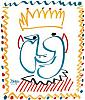# Coding help - page 2821285

Hello guys and gurus,

Have you code snippet, for a customizable money management which evolves with Balance, that i could incorporate into an ea ?

Thanks !230423

Big Joe:
Hello guys and gurus,

Have you code snippet, for a customizable money management which evolves with Balance, that i could incorporate into an ea ?

Thanks !

Joe

Why don't you use equity instead of balance?1285

Joe Why don't you use equity instead of balance?

Ah yes, sorry i was wrong !

Equity of course !230423

Big Joe:
Ah yes, sorry i was wrong ! Equity of course !

Joe

Try out this one : lot_size.mq4

It is made as an indicator, so you can test it on chart to see what results are you going to get for various stop losses and risk %. It uses account free margin for calculation, but you can easily replace that with a function of your choice

Files:
lot_size.mq4  2 kb1285

Joe

Try out this one : lot_size.mq4

It is made as an indicator, so you can test it on chart to see what results are you going to get for various stop losses and risk %. It uses account free margin for calculation, but you can easily replace that with a function of your choice

Thank you masterI work on an arbitrage ea.

Can you tell me, if the code bellow, will calculate the profit with the orders with the same MagicNumber and so will close only trades with same MagicNumber ?

Thank you !

double getTotalProfits()

{

int total = OrdersTotal();

double profits = 0.0;

for(int cnt = 0;cnt < total;cnt++)

{

if (OrderMagicNumber() == MagicNumber)

{

profits += OrderProfit();

}

}

return(profits);

}

bool profitTargetReached()

{

if (getTotalProfits() >= ((AccountBalance() * (PercentTakeProfit / 100.0))))

{

return(true);

}

return(false);230423

Big Joe:
Thank you masterI work on an arbitrage ea.

Can you tell me, if the code bellow, will calculate the profit with the orders with the same MagicNumber and so will close only trades with same MagicNumber ?

Thank you !

double getTotalProfits()

{

int total = OrdersTotal();

double profits = 0.0;

for(int cnt = 0;cnt < total;cnt++)

{

if (OrderMagicNumber() == MagicNumber)

{

profits += OrderProfit();

}

}

return(profits);

}

bool profitTargetReached()

{

if (getTotalProfits() >= ((AccountBalance() * (PercentTakeProfit / 100.0))))

{

return(true);

}

return(false);

Joe,

Yes. What you can add (to be 100% accurate) is these two parts two that can influence the profit :

profits += (OrderProfit()+OrderSwap()+OrderCommission());1285

Joe,

Yes. What you can add (to be 100% accurate) is these two parts two that can influence the profit :

profits += (OrderProfit()+OrderSwap()+OrderCommission());

OK !

Thank you for your time MladenI'm learning day after day.1366

I have a txt file with signals like:

May 01, 2014 at 07:53AM Gold OP_BUYSTOP 1292.4; Stop Loss 1265.2; Profit Target 1358.4

May 01, 2014 at 06:52AM Silver OP_SELL 1961.5; Stop Loss 2030.5; Profit Target 1855

So have any of you an idea how to test this signal? So I can see if this signals where good or bad...[Deleted]

Mr Mladen, i was wrong, what i need is to filter all trades, i have tested and works good with Stochastic (5,3,3), if higher than 80, and actual signal is for sell, then sell. If lower than 20, and actual signal is for buy, then buy. If not, don't open orders at this entry point.

THank you

Files:165